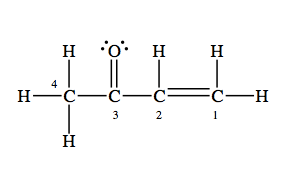# Problem: Consider the following Lewis structure below and select which statement is false.a) there are 10 sigma and 2 pi bondsb) the C-2 atom is sp2 hybridized with bond angles of about 120°c) the oxygen atom is sp3 hybridizedd) this molecule contains 28 valence electronse) there are some H—C—H bond angles of about 109° in the molecule

###### FREE Expert Solution
100% (230 ratings)
###### Problem Details

Consider the following Lewis structure below and select which statement is false.

a) there are 10 sigma and 2 pi bonds

b) the C-2 atom is sp2 hybridized with bond angles of about 120°

c) the oxygen atom is sp3 hybridized

d) this molecule contains 28 valence electrons

e) there are some H—C—H bond angles of about 109° in the moleculeFrequently Asked Questions

What scientific concept do you need to know in order to solve this problem?

Our tutors have indicated that to solve this problem you will need to apply the Hybridization concept. You can view video lessons to learn Hybridization. Or if you need more Hybridization practice, you can also practice Hybridization practice problems.

What is the difficulty of this problem?

Our tutors rated the difficulty ofConsider the following Lewis structure below and select whic...as low difficulty.

How long does this problem take to solve?

Our expert Chemistry tutor, Jules took 2 minutes and 19 seconds to solve this problem. You can follow their steps in the video explanation above.

What professor is this problem relevant for?

Based on our data, we think this problem is relevant for Professor Robinson's class at UGA.